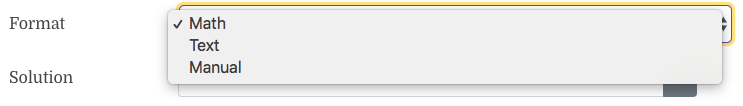# What does "format" mean for a problem?

Q: What does “format” mean for a problem?

A: The format selection exists for questions created prior to February 21st, 2019. It determines how the solution is shown. Math mode allows you to write math expressions such as fractions, exponents, integrals, symbols, and more. The text mode should be used when there are no mathematical expressions in the answer. The manual mode should be used when the answer does not have an exact answer, or answers, and you would like to view the answer submitted to grade the problem (i.e. graphs, descriptions, and more)